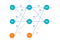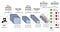# Creating a simple convolution neural network

In this three-part series, we will create a machine learning model that can classify different “natural scenes”. We will be using the Intel Image Classification dataset from Kaggle.

In this blog post, we will talk about object detection, take a look at our data, and understand and create a simple convolution neural network to classify six different “natural scenes.”

# Object detection

Object localization is locating the presence of objects in an image and finding a bounding box for that object. Object recognition is classifying the objects that the model found.

# Understanding and preprocessing the data

Here is my Kaggle Notebook if you would like to follow along: https://www.kaggle.com/krish45732/intel-image-classification-notebook. (The output of the notebook and the output on this blog post may be different)

We will start by importing libraries that we will need later on

The data is separated into six folders: one for each class. We will make a `get_images` function to extract our images into one array. First, we make empty lists to store the images (`x_list`) and their labels (`y_list`). Next, the outer loop will cycle through each class (0–6). The inner loop will cycle through each image in the classes’ respective folders. We will use the cv2 library to read the images, resize them to (150, 150, 3) and append them to `x_list`. We also append the class number to the `y_list` variable. Lastly, we convert the python lists into NumPy arrays, shuffle the data, and return the arrays. The `get_images_pred` is similar to the function we just made, but it has only one loop and doesn’t have `y_list`. The `to_onehot` function will be used later to convert the y values to a one-hot array.

Next, we create a dictionary to hold a mapping from the number of the class to its name. Then, we use the `get_images` function to get the training and validation images. The validation dataset will be used to check if our model is overfitting or “memorizing” the training dataset.

Next, we will visualize the data using matplotlib. This lets us confirm that we got the right images with the right classes and preview the images we will be using.

The output images will vary since we shuffled the images before, but confirm that the labels match their images. Here is my output:

Now that we have taken a look at our dataset, we can get started with creating our model. First, we convert our label arrays into one-hot arrays using the `to_onehot` function we previously made.

# Artificial neural networks (ANN)Image from missinglink.ai

# Convolution neural networks (CNN)Image from towardsdatascience.com

A convolution neural network is an algorithm commonly used in object detection or object recognition. CNN’s usually perform better on images compared to regular perceptrons. It essentially reduces the image size while maintaining the image’s key features to make processing easier. As shown below, convolution neural networks can have convolution layers, max-pooling layers, flatten layer, dense/fully connected layers, and more.

In the GIF on the left, the leftmost image is the input; the middle image is the convolution filter, and the rightmost image is the output. In this example, the 3x3 filter is multiplied onto a 3x3 section of the image. The values are summed up and put in the output image. Some things that can change include the filter size, the stride (how many pixels the filter moves by), and padding (which can keep the image the same size). To learn more about convolution layers you can read this article.

Max-pool layers downsample the image into key features reducing the computation power needed. The max-pool layer takes the max value using a filter. The flatten layer takes the output from the last convolution/pooling layer and flattens it down into a one-dimensional array to be used in the dense layers.

# Analyzing the results

Note that the graph will vary. Using the graph, we can see that our training accuracy and validation accuracy increased a lot in the first few epochs. Near the end, the training accuracy was still going up but started to flatten out. However, the validation accuracy was going down. This is called overfitting in which the model starts to memorize the training set. So, the training set accuracy will go up, but the validation set accuracy, which is not being trained on, will go down. According to the print statement and the graph, epoch 15 was the best epoch with a validation accuracy of 83.33%. In future articles, we will talk about how to reduce overfitting and increase accuracy.

Now, we can use our model to predict images it has not seen before. First, we load the prediction dataset using the `get_images_pred` function we made before. Then we use the `predict_classes` function from Keras to predict the classes. Lastly, we plot 16 images from the prediction set with their labels.

The output can vary but compare the labels to what you think that image is. Here is my output:

# Conclusion

Krish Ranjan is a Student Ambassador in the Inspirit AI Student Ambassadors Program. Inspirit AI is a pre-collegiate enrichment program that exposes curious high school students globally to AI through live online classes. Learn more at https://www.inspiritai.com/.